CATEGORIES:

# MAIN METHODS OF INTEGRATION

Integration by substitution ( or change of variable )

Steps for Integrating by Substitution—Indefinite Integrals:

1. Choose a substitution u = g(x), such as the inner part of a composite function.

2. Compute.

3. Re-write the integral in terms of u and du.

4. Find the resulting integral in terms of u.

5. Substitute g(x) back in for u, yielding a function in terms of x only.

6. Check by differentiating.

If f(x) is continous function, F(x)- its antiderivative and φ(х)- differentiable function, then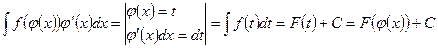In the particular case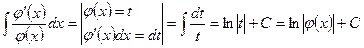Example 8. To find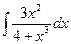. Notice that the numerator is the derivative of the denominator

Let. Differentiating gives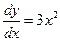and hence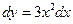.

Substituting this change of variable the integral becomesNow by expressing this result in terms of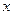we have shown that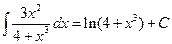.

Integration by parts

By the Product Rule for Derivatives,. Thus,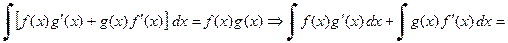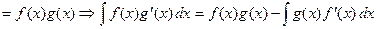. This formula for integration

by parts often makes it possible to reduce a complicated integral involving a product to

a simpler integral. By letting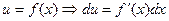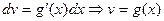we get the more common formula for integration by parts: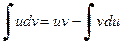.

Example 9. Find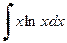.

Letand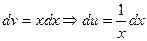and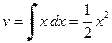. Thus,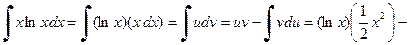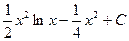.

It is possible that when you set up an integral using integration by parts, the resulting

integral will be more complicated than the original integral. In this case, change your

substitutions for u and dv.

Date: 2015-01-02; view: 1592

doclecture.net - lectures - 2014-2023 year. Copyright infringement or personal data (0.016 sec.)